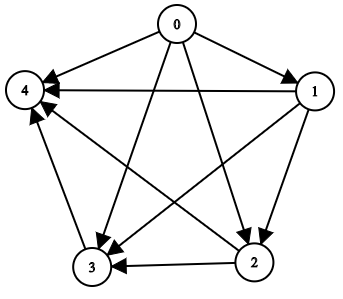##### Welcome to Subscribe On Youtube

Formatted question description: https://leetcode.ca/all/2192.html

# 2192. All Ancestors of a Node in a Directed Acyclic Graph (Medium)

You are given a positive integer n representing the number of nodes of a Directed Acyclic Graph (DAG). The nodes are numbered from 0 to n - 1 (inclusive).

You are also given a 2D integer array edges, where edges[i] = [fromi, toi] denotes that there is a unidirectional edge from fromi to toi in the graph.

Return a list answer, where answer[i] is the list of ancestors of the ith node, sorted in ascending order.

A node u is an ancestor of another node v if u can reach v via a set of edges.

Example 1:Input: n = 8, edgeList = [[0,3],[0,4],[1,3],[2,4],[2,7],[3,5],[3,6],[3,7],[4,6]]
Output: [[],[],[],[0,1],[0,2],[0,1,3],[0,1,2,3,4],[0,1,2,3]]
Explanation:
The above diagram represents the input graph.
- Nodes 0, 1, and 2 do not have any ancestors.
- Node 3 has two ancestors 0 and 1.
- Node 4 has two ancestors 0 and 2.
- Node 5 has three ancestors 0, 1, and 3.
- Node 6 has five ancestors 0, 1, 2, 3, and 4.
- Node 7 has four ancestors 0, 1, 2, and 3.


Example 2:Input: n = 5, edgeList = [[0,1],[0,2],[0,3],[0,4],[1,2],[1,3],[1,4],[2,3],[2,4],[3,4]]
Output: [[],,[0,1],[0,1,2],[0,1,2,3]]
Explanation:
The above diagram represents the input graph.
- Node 0 does not have any ancestor.
- Node 1 has one ancestor 0.
- Node 2 has two ancestors 0 and 1.
- Node 3 has three ancestors 0, 1, and 2.
- Node 4 has four ancestors 0, 1, 2, and 3.


Constraints:

• 1 <= n <= 1000
• 0 <= edges.length <= min(2000, n * (n - 1) / 2)
• edges[i].length == 2
• 0 <= fromi, toi <= n - 1
• fromi != toi
• There are no duplicate edges.
• The graph is directed and acyclic.

Companies:

Related Topics:
Depth-First Search, Breadth-First Search, Graph, Topological Sort

Similar Questions:

## Solution 1. DFS

// OJ: https://leetcode.com/problems/all-ancestors-of-a-node-in-a-directed-acyclic-graph/
// Time: O(N^2)
// Space: O(N^2)
class Solution {
public:
vector<vector<int>> getAncestors(int n, vector<vector<int>>& E) {
vector<vector<int>> G(n), ans(n);
bool connect = {};
for (auto &e : E) G[e].push_back(e);
function<void(int, int)> dfs = [&](int u, int s) {
if (connect[s][u]) return; // If s has been added as a source node of u already, skip
connect[s][u] = true;
for (int v : G[u]) dfs(v, s);
};
for (int i = 0; i < n; ++i) dfs(i, i);
for (int i = 0; i < n; ++i) {
for (int j = 0; j < n; ++j) {
if (i != j && connect[i][j]) ans[j].push_back(i);
}
}
return ans;
}
};


## Solution 2.

// OJ: https://leetcode.com/problems/all-ancestors-of-a-node-in-a-directed-acyclic-graph/
// Time: O(N^2)
// Space: O(E)
class Solution {
public:
vector<vector<int>> getAncestors(int n, vector<vector<int>>& E) {
vector<vector<int>> G(n), ans(n);
for (auto &e : E) G[e].push_back(e);
function<void(int, int)> dfs = [&](int u, int s) {
for (int v : G[u]) {
if (ans[v].size() && ans[v].back() == s) continue;
ans[v].push_back(s);
dfs(v, s);
}
};
for (int i = 0; i < n; ++i) dfs(i, i);
return ans;
}
};